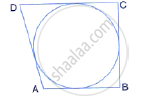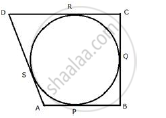Share

# If the Sides of a Quadrilateral Abcd Touch a Circle, Prove That: Ab + Cd = Bc + Ad - Mathematics

Course

#### Question

If the sides of a quadrilateral ABCD touch a circle, prove that:
AB + CD = BC + AD#### SolutionLet the circle touch the sides AB, BC, CD and DA of quadrilateral ABCD at P, Q, R and S respectively.
Since AP and AS are tangents to the circle from external point A
AP = AS .......(i)
Similarly, we can prove that:
BP = BQ .......(ii)
CR = CQ .......(iii)
DR = DS ........(iv)
AP + BP + CR + DR = AS + DS + BQ + CQ
AB + CD = AD + BC
Hence, AB + CD = AD + BC

Is there an error in this question or solution?